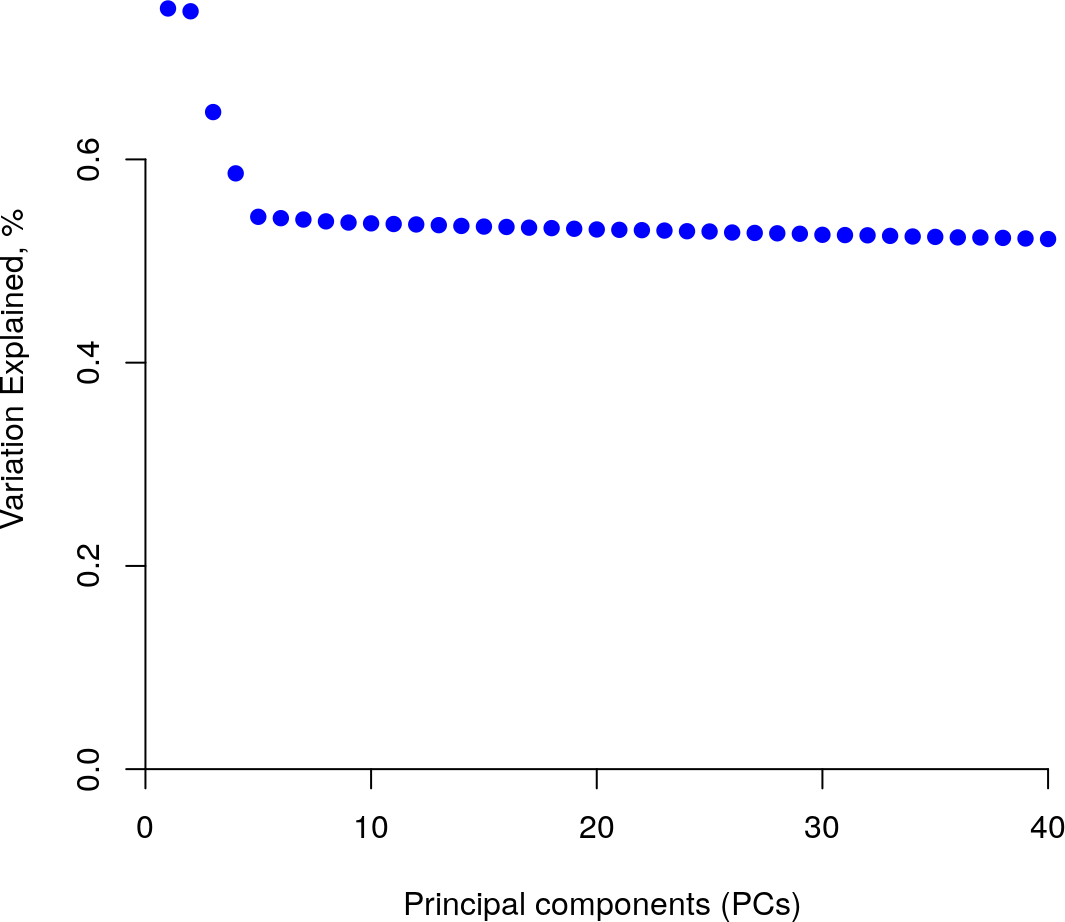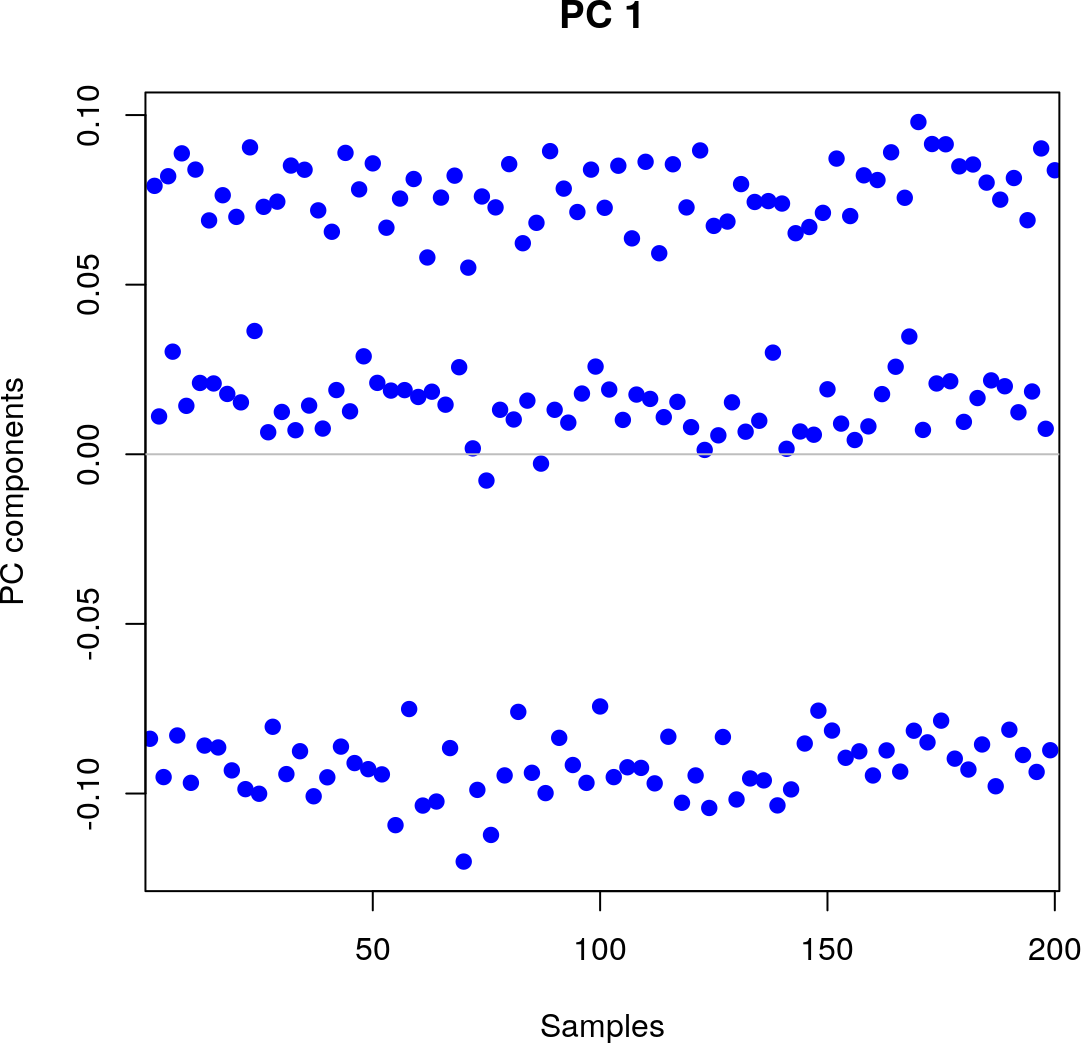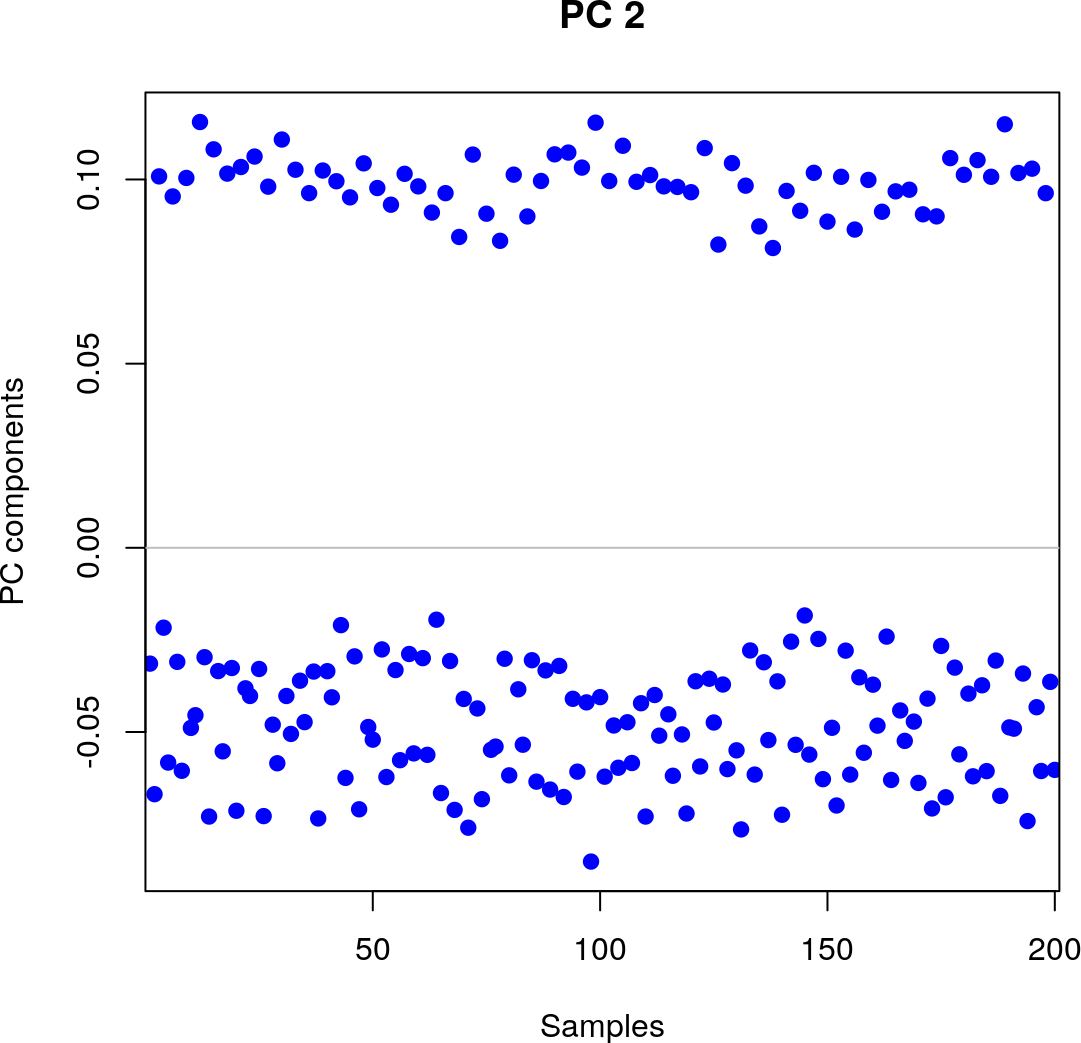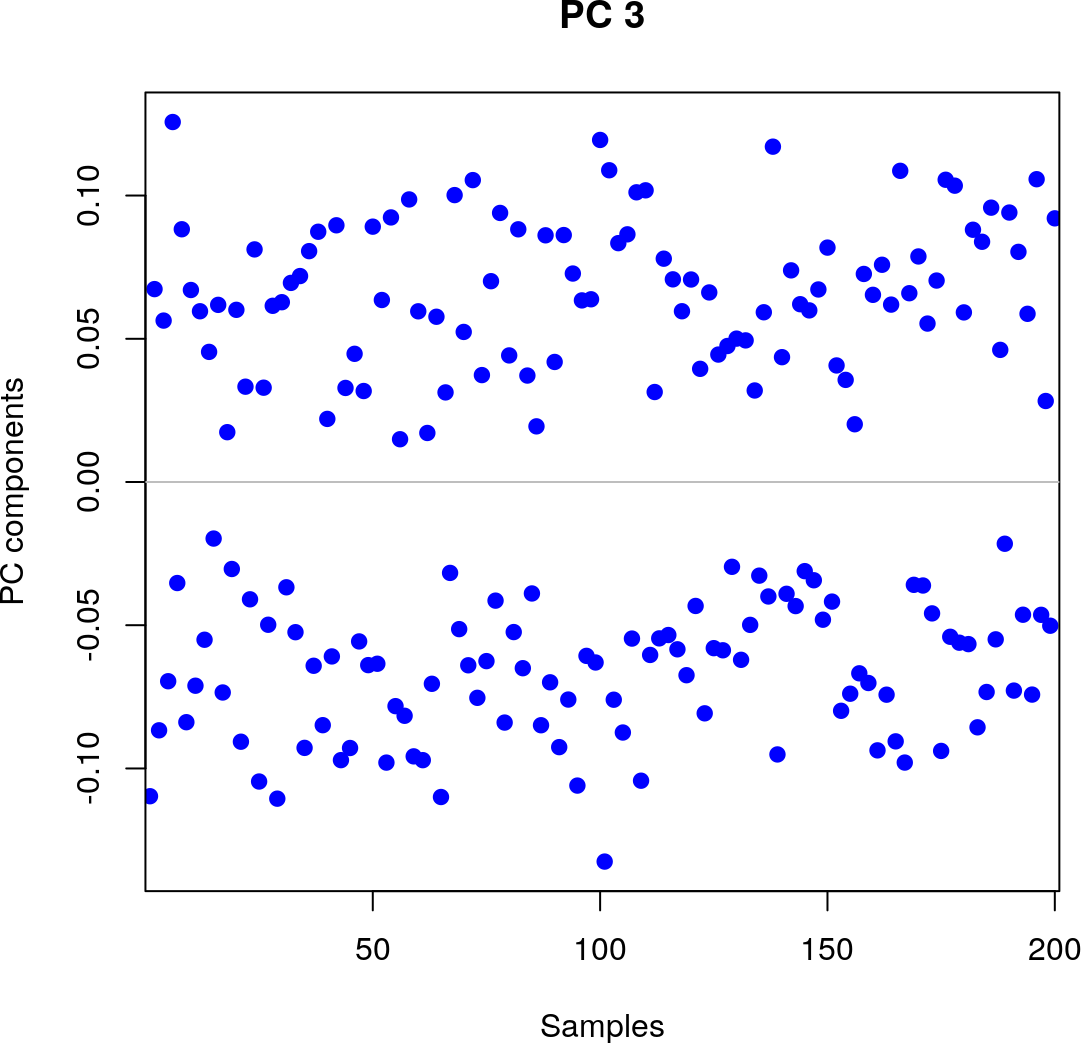# 1 Using RaMWAS with other methylation platforms or data types

RaMWAS is primarily designed for studies of methylation measurements from enrichment platforms.

However, RaMWAS can also be useful for the analysis of methylation measurements from other platforms (e.g. Illumina HumanMethylation450K array) or other data types such as gene expression levels or genotype information. RaMWAS can perform several analysis steps on such data including: principal component analysis (PCA), association testing (MWAS, TWAS, GWAS), and multimarker analysis with cross validation using the elastic net.

## 1.1 Import data from other sources

Without external data source at hand, we show how to create and fill data matrices with artificial data. Importing real data can be done in a similar way, with random data generation replaced with reading data from existing sources.

We create data files in the same format as produced by Step 3 of RaMWAS.

These files include

• `CpG_locations.*` – filematrix with the location of the CpGs / SNPs / gene trascription start sites.
It must have two columns with integer values – chromosome number and location (`chr` and `position`).
• `CpG_chromosome_names.txt` – file with chromosome names (factor levels) for the integer column `chr` in the location filematrix.
• `Coverage.*` – filematrix with the data for all samples and all locations.
Each row has data for a single sample. Row names must be sample names.
Each column has data for a single location (CpG / SNP / gene trascription start site). Columns must match rows of the location filematrix.

First, we load the package and set up a working directory. The project directory `dr` can be set to a more convenient location when running the code.

``````library(ramwas)

# work in a temporary directory
dr = paste0(tempdir(), "/simulated_matrix_data")
dir.create(dr, showWarnings = FALSE)
cat(dr,"\n")``````
``## /tmp/RtmpwHjGHT/simulated_matrix_data``

Let the sample data matrix have 200 samples and 100,000 variables.

``````nsamples = 200
nvariables = 100000``````

For these 200 samples we generate a data frame with age and sex phenotypes and a batch effect covariate.

``````covariates = data.frame(
sample = paste0("Sample_",seq_len(nsamples)),
sex = seq_len(nsamples) %% 2,
age = runif(nsamples, min = 20, max = 80),
batch = paste0("batch",(seq_len(nsamples) %% 3))
)
sample sex age batch
Sample_1 1 71.5 batch1
Sample_2 0 35.8 batch2
Sample_3 1 60.4 batch0
Sample_4 0 64.5 batch1
Sample_5 1 28.4 batch2
Sample_6 0 26.3 batch0

Next, we create the genomic locations for 100,000 variables.

``````temp = cumsum(sample(20e7 / nvariables, nvariables, replace = TRUE) + 0)
chr      = as.integer(temp %/% 1e7) + 1L
position = as.integer(temp %% 1e7)

locmat = cbind(chr = chr, position = position)
chrnames = paste0("chr", 1:10)
chr position
1 958
1 1850
1 2916
1 4390
1 5386
1 6104

Now we save locations in a filematrix and create a text file with chromosome names.

``````fmloc = fm.create.from.matrix(
filenamebase = paste0(dr,"/CpG_locations"),
mat = locmat)
close(fmloc)
writeLines(
con = paste0(dr,"/CpG_chromosome_names.txt"),
text = chrnames)``````

Finally, we create data matrix. We include sex effect in 225 variables and age effect in 16 variables out of each 2000. Each variable is also affected by noise and batch effects.

``````fm = fm.create(paste0(dr,"/Coverage"), nrow = nsamples, ncol = nvariables)

# Row names of the matrix are set to sample names
rownames(fm) = as.character(covariates\$sample)

# The matrix is filled, 2000 variables at a time
byrows = 2000
for( i in seq_len(nvariables/byrows) ){ # i=1
slice = matrix(runif(nsamples*byrows), nrow = nsamples, ncol = byrows)
slice[,  1:225] = slice[,  1:225] + covariates\$sex / 30 / sd(covariates\$sex)
slice[,101:116] = slice[,101:116] + covariates\$age / 10 / sd(covariates\$age)
slice = slice + ((as.integer(factor(covariates\$batch))+i) %% 3) / 40
fm[,(1:byrows) + byrows*(i-1)] = slice
}
close(fm)``````

## 1.2 Principal Component Analysis (PCA)

To run PCA with RaMWAS we specify three parameters:

• `dircoveragenorm` – directory with the data matrix
• `covariates` – data frame with covariates
• `modelcovariates` – names of covariates to regress out
``````param = ramwasParameters(
dircoveragenorm = dr,
covariates = covariates,
modelcovariates = NULL
)``````

Now we run PCA.

``ramwas4PCA(param)``

The top several PCs are marginally distinct from the rest.

``````pfull = parameterPreprocess(param)
eigenvectors = fm.open(
filenamebase = paste0(pfull\$dirpca, "/eigenvectors"),
plotPCvalues(eigenvalues)````````plotPCvectors(eigenvectors[,1], 1)````plotPCvectors(eigenvectors[,2], 2)````plotPCvectors(eigenvectors[,3], 3)````plotPCvectors(eigenvectors[,4], 4)``